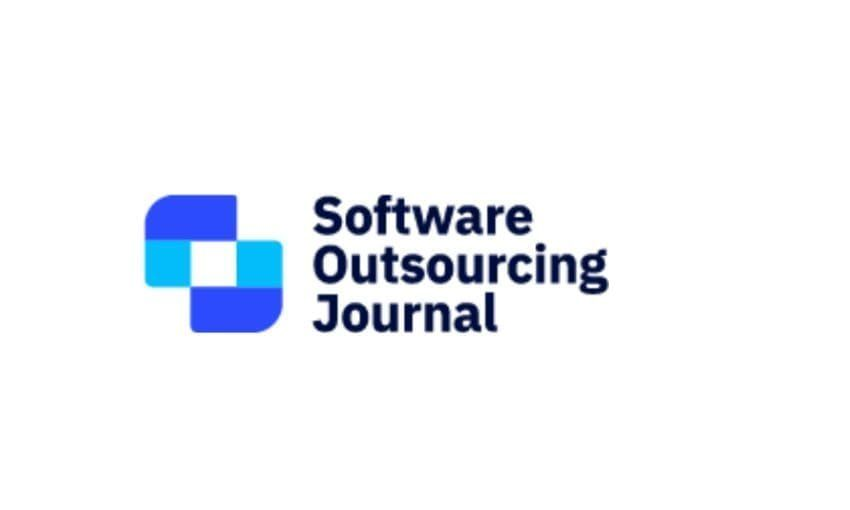# Daniel Rogozin Participates in BLAST 2021

Our logician Daniel Rogozin recently participated in BLAST 2021 (an abbreviation for Boolean Algebras, Lattices, Algebraic and Quantum logic, Universal Algebra, Set Theory Set-theoretic and Point-free Topology). This conference was held at the Zoom version of the New Mexico State University.

Daniel’s talk was about algebraic logic. In particular, he’s interested in relation algebras, the kind of Boolean algebras with operations that provide an approximate algebraisation of binary relations.

## Background

When we study algebraic structures, we often investigate the questions of representability, that is, possible realisations of those algebras as ‘less’ abstract structures. There are two canonical examples of representations. The first is Cayley’s theorem, which states that every group $G$ is isomorphic to the subgroup of the symmetric group on $G$. The second example is Stone’s theorem, according to which every Boolean algebra is isomorphic to some algebra of clopens of a suitable compact Hausdorff zero-dimensional topological space.

The problem is that we don’t have the uniform representation theorem for relation algebras. The first examples of non-representable relation algebras are due to Roger Lyndon. That is, there are relation algebras that cannot be realised as set algebras of binary relations. The second problem is that representability is undecidable for finite relation algebras. In other words, no algorithm would determine whether a given relation algebra is representable or not. This negative result is due to Robin Hirsch and Ian Hodkinson. For these reasons, reducts (a restriction of a structure to a subset of operations) of relation algebras are of interest. Reducts might be a bit ‘better’ from an algorithmic point of view, and some of them might have the uniform representation theorem.

The aspect of relation algebra reduct we are interested in is the finite representation property. A class of representable reducts has the finite representation property if every finite member of this class is representable over a finite base, that is, all those binary relations can be defined on some finite domain. This property has a fruitful implication. If a given class has the FRP and a recursively enumerable axiomatisation, then the representability problem is decidable for finite representable structures.

## The results acquired

The first class of relation algebra reducts that Daniel discussed in his talk was the class of representable residuated semigroups. These algebras are of interest for specialists in such substructural logics as the Lambek calculus. This logic is a formalisation of the notion of an inference in categorial grammars, the equivalent version of context-free ones. We already know that this class is finitely axiomatisable. Finite representability was the issue, see Problem 19.17 in the book by Hodkinson and Hirsch called ‘Relation algebras by games’. Daniel has managed to find a positive solution for this problem, and the argument is surprisingly simple. One needs to observe that any residuated semigroup $R$ is isomorphic to the subalgebra of a quantale of Galois stable subsets $R$, this is due to Robert Goldblatt. Recall that a quantale is a complete lattice-ordered semigroup, i.e. a partially ordered semigroup such that every subset has the least upper bound. But every quantale is representable with binary relations. See this paper by Brown and Gurr. The combination of these two results solves the problem by Hirsch and Hodkinson positively. Daniel’s contribution is an observation that such a combination does exist.

The second kind of algebras considered in this talk was the class of representable join semilattice-ordered semigroups. There exist non-representable join semilattice-ordered semigroups whose non-principal ultraproduct is representable, so this class is not finitely axiomatisable, the example is due to Andreka and Mikulas. So Daniel characterised representability using so-called back-and-forth games. The reader may have a look at this introductory lecture by Wilfrid Hodges about games in model theory to have a basic understanding of such techniques. Daniel also proved that the class of representable join semilattice-ordered semigroups has a recursively enumerable axiomatisation. The finite representation property for this class remains the issue. Daniel already had a couple of attempts, but all of them have happened to be broken for several reasons.

Daniel Rogozin Participates in BLAST 2021More from Serokell